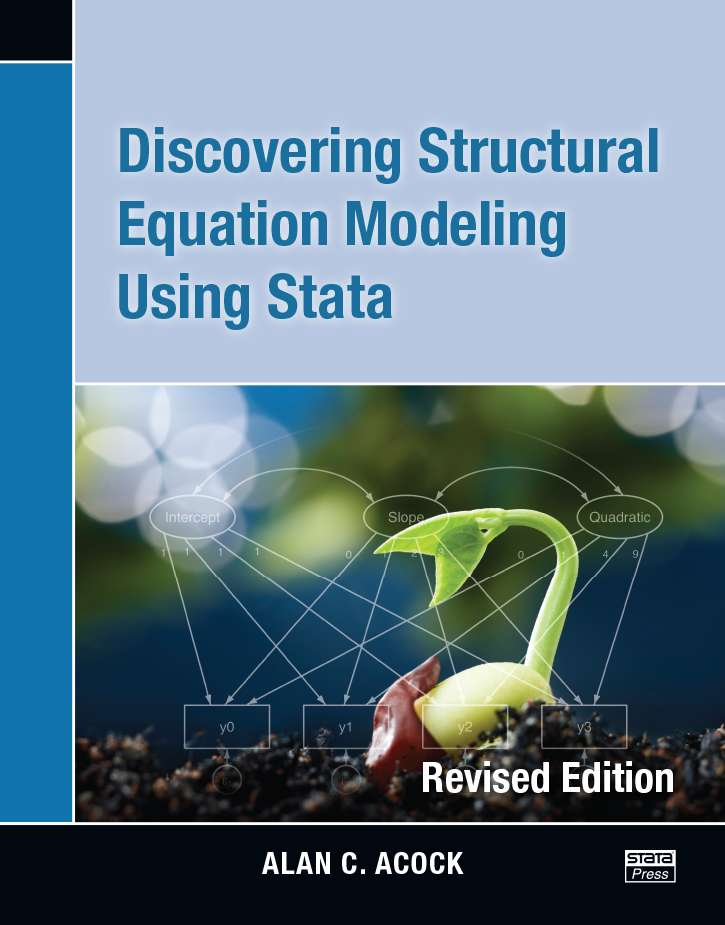# Discovering Structural Equation Modeling Using Stata

Discovering Structural Equation Modeling Using Stata, by Alan Acock, successfully introduces both the statistical principles involved in structural equation modeling (SEM) and the use of Stata to fit these models. The book uses an application-based approach to teaching SEM. Acock demonstrates how to fit a wide variety of models that fall within the SEM framework and provides datasets that enable the reader to follow along with each example. As each type of model is discussed, concepts such as identification, handling of missing data, model evaluation, and interpretation are covered in detail.

In Stata, structural equation models can be fit using the command language or the graphical user interface (GUI) for SEM, known as the SEM Builder. The book demonstrates both of these approaches. Throughout the text, the examples use the semcommand. Each chapter also includes brief discussions on drawing the appropriate path diagram and performing estimation from in the SEM builder. A more in-depth coverage of the SEM Builder is given in one of the book’s appendixes.

The first two chapters introduce the building blocks of SEM. Chapter 1 begins with overviews of Chronbach’s alpha as a measure of reliability and of exploratory factor analysis. Then, building on these concepts, Acock demonstrates how to perform confirmatory factor analysis, discusses a variety of statistics available for assessing the fit of the model, and shows a more general measurement of reliability that is based on confirmatory factor analysis. Chapter 2 focuses on using SEM to perform path analysis. It includes examples of mediation, moderation, cross-lagged panel models, and nonrecursive models.

Chapter 3 demonstrates how to combine the topics covered in the first two chapters to fit full structural equation models. The use of modification indices to guide model modification and computation of direct, indirect, and total effects for full structural equation models are also covered.

Chapter 4 details the application of SEM to growth curve modeling. After introducing the basic linear latent growth curve model, Acock extends this to more complex cases such as the inclusion of quadratic terms, time-varying covariates, and time-invariant covariates.

Chapter 5 discusses testing for differences across groups in SEM. The author introduces the specialized sem syntax for multiple group models and discusses the intricacies of testing for group differences for the different types of models presented in the preceding chapters.

Discovering Structural Modeling Using Stata is an excellent resource both for those who are new to SEM and for those who are familiar with SEM but new to fitting these models in Stata. It is useful as a text for courses covering SEM as well as for researchers performing SEM.

List of Tables
List of Figures
List of examples
Preface
Acknowledgements

1. INTRODUCTION TO CONFIRMATORY FACTOR ANALYSIS

Introduction
The “do not even think about it” approach
The principal component factor analysis approach
Alpha reliability for our nine-item scale
Generating a factor score rather than a mean or summative score
Fitting a CFA model
Interpreting and presenting CFA results
Assessing goodness of fit

Modification indices
Final model and estimating scale reliability

A two-factor model

Evaluating the depression dimension
Estimating a two-factor model

Parceling
Extensions and what is next
Exercises
Using the SEM Builder to run a CFA

Drawing the model
Estimating the model

2. USING STRUCTURAL EQUATION MODELING FOR PATH MODELS

Introduction
Path model terminology

Exogenous predictor, endogenous outcome, and endogenous mediator variables
A hypothetical path model

A substantive example of a path model
Estimating a model with correlated residuals

Estimating direct, indirect, and total effects
Strengthening our path model and adding covariates

Auxiliary variables
Testing equality of coefficients
A cross-lagged panel design
Moderation
Nonrecursive models

Worked example of a nonrecursive model
Stability of a nonrecursive model
Model constraints
Equality constraints

Exercises
Using the SEM Builder to run path models

The probit model

3. STRUCTURAL EQUATION MODELING

Introduction
The classic example of a structural equation model

Identification of a full structural equation model
Fitting a full structural equation model
Modifying our model
Indirect effects

Equality constraints
Programming constraints
Structural model with formative indicators

Identification and estimation of a composite latent variable
Multiple indicators, multiple causes model

Exercises

4. LATENT GROWTH CURVES

Discovering growth curves
A simple growth curve model
Identifying a growth curve model

An intuitive idea of identification

An example of a linear latent growth curve

A latent growth curve model for BMI
Graphic representation of individual trajectories (optional)
Intraclass correlation (ICC) (optional)
Fitting a latent growth curve

How can we add time-invariant covariates to our model?

Interpreting a model with time-invariant covariates

Explaining the random effects—time-varying covariates

Fitting a model with time-invariant and time-varying covariates
Interpreting a model with time-invariant and time-varying covariates

Constraining variances of error terms to be equal (optional)
Exercises

5. GROUP COMPARISONS

Interaction as a traditional approach to multiple-group comparisons
The range of applications of Stata’s multiple-group comparisons with sem

A multiple indicators, multiple causes model
A measurement model
A full structural equation model

A measurement model application

Step 1: Testing for invariance comparing women and men
Testing for equal intercepts
Comparison of models
Step 4: Comparison of means
Step 5: Comparison of variances and covariance of latent variables

Multiple-group path analysis

What parameters are different?
Fitting the model with the SEM Builder
A standardized solution
Constructing tables for publications

Multiple-group comparisons of structural equation models
Exercises

6. EPILOGUE- WHAT NOW?

A. THE GRAPHICAL USER INTERFACE

Introduction
Menus for Windows, Unix, and Mac

The vertical drawing toolbar

Designing a structural equation model
Drawing an SEM model
Fitting a structural equation model
Postestimation commands
Clearing preferences and restoring the defaults

B. ENTERING DATA FROM SUMMARY STATISTICSAuthor: Alan C. Acock
Edition: Revised Edition
ISBN-13: 978-1-59718-139-6